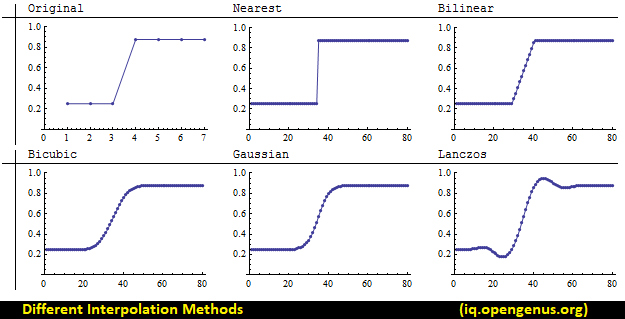# Different Interpolation methods in OpenCV

Get FREE domain for 1st year and build your brand new siteIn this article, we have presented the 10 different Interpolation methods in OpenCV library. It is useful in resizing images using OpenCV.

1. Interpolation in OpenCV
2. INTER_NEAREST interpolation in OpenCV
3. INTER_LINEAR
4. INTER_LINEAR_EXACT
5. INTER_AREA
6. INTER_CUBIC
7. INTER_LANCZOS4
8. INTER_NEAREST_EXACT
9. INTER_MAX
10. WARP_FILL_OUTLIERS
11. WARP_INVERSE_MAP

## Interpolation in OpenCV

Interpolation is the way the extra pixels in the new image is calculated. If the original image is smaller, then a larger rescaled image has extra pixels which is not exactly the same as a nearby pixels.

The value of the extra pixel depends on the technique used. The different interpolation techniques used in OpenCV are:

• INTER_NEAREST: nearest neighbor interpolation technique
• INTER_LINEAR: bilinear interpolation (default)
• INTER_LINEAR_EXACT
• INTER_AREA: resampling using pixel area relation
• INTER_CUBIC: bicubic interpolation over 4 x 4 pixel neighborhood
• INTER_LANCZOS4: Lanczos interpolation over 8 x 8 pixel neighborhood
• INTER_NEAREST_EXACT
• INTER_MAX
• WARP_FILL_OUTLIERS
• WARP_INVERSE_MAP

In OpenCV Python, Interpolation method is used while resizing an image as follows:

``````image = cv2.resize(image, dsize=(new_height, new_width),
interpolation=cv2.INTER_CUBIC)
``````

This image gives an idea of how images are interpolated using different methods:## INTER_NEAREST interpolation in OpenCV

This option uses the nearest neighbor interpolation algorithm. It retains the sharpness of the edges though the overall image may be blurred.

It is used as follows:

``````image = cv2.resize(image, dsize=(new_height, new_width),
interpolation=cv2.INTER_NEAREST)
``````

## INTER_LINEAR

This option uses the bilinear interpolation algorithm. Unlike INTER_NEAREST, this does the interpolation in two dimensions and predicts the function used to calculate the color of a pixel.

This algorithm is effective in handling visual distortions while zooming or enlarging an image.

It is used as follows:

``````image = cv2.resize(image, dsize=(new_height, new_width),
interpolation=cv2.INTER_LINEAR)
``````

## INTER_LINEAR_EXACT

INTER_LINEAR_EXACT is a modification of INTER_LINEAR and both uses bilinear interpolation algorithm. The only difference is that the calculations in INTER_LINEAR_EXACT are accurate to a bit.

It is used as follows:

``````image = cv2.resize(image, dsize=(new_height, new_width),
interpolation=cv2.INTER_LINEAR_EXACT)
``````

## INTER_AREA

This option uses resampling using pixel area relation technique. While enlarging images, INTER_AREA work same as INTER_NEAREST.

In other cases, INTER_AREA works better in image decimation and avoiding false inference patterns in images (moire pattern).

It is used as follows:

``````image = cv2.resize(image, dsize=(new_height, new_width),
interpolation=cv2.INTER_AREA)
``````

## INTER_CUBIC

This option uses bicubic interpolation technique. This is an extension of cubic interpolation technique and is used for 2 dimension regular grid patterns.

It is used as follows:

``````image = cv2.resize(image, dsize=(new_height, new_width),
interpolation=cv2.INTER_CUBIC)
``````

## INTER_LANCZOS4

This option uses Lanczos interpolation over 8 x 8 pixel neighborhood technique. It uses Fourier series and Chebyshev polynomials and is suited for images with large number of small size details.

It is used as follows:

``````image = cv2.resize(image, dsize=(new_height, new_width),
interpolation=cv2.INTER_LANCZOS4)
``````

## INTER_NEAREST_EXACT

INTER_NEAREST_EXACT is a modification of INTER_NEAREST with bit level accuracy.

It is used as follows:

``````image = cv2.resize(image, dsize=(new_height, new_width),
interpolation=cv2.INTER_NEAREST_EXACT)
``````

## INTER_MAX

This option uses mask for interpolation codes.

It is used as follows:

``````image = cv2.resize(image, dsize=(new_height, new_width),
interpolation=cv2.INTER_MAX)
``````

## WARP_FILL_OUTLIERS

This interpolation technique skips the outliers during interpolation calculations.

It is used as follows:

``````image = cv2.resize(image, dsize=(new_height, new_width),
interpolation=cv2.WARP_FILL_OUTLIERS)
``````

## WARP_INVERSE_MAP

This option uses inverse transformation technique for interpolation.

It is used as follows:

``````image = cv2.resize(image, dsize=(new_height, new_width),
interpolation=cv2.WARP_INVERSE_MAP)
``````

With this article at OpenGenus, you must have the complete idea of Different Interpolation methods in OpenCV.